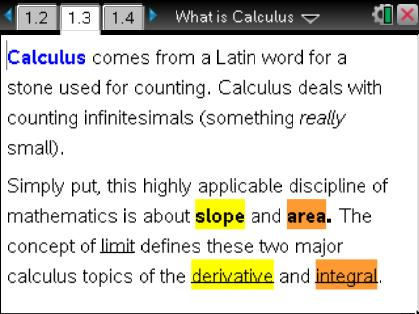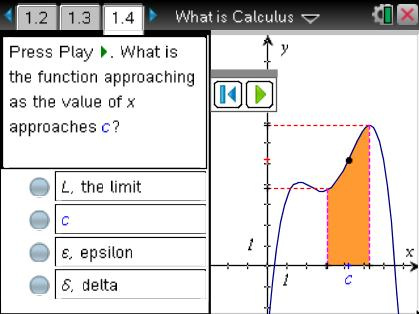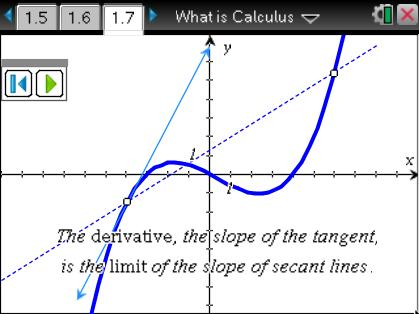# Activities

••• ##### Subject Area

• Math: Calculus: Applications of the Derivative
• Math: Calculus: Derivatives
• Math: Calculus: Antiderivatives and Slope Fields
• Math: Calculus: Limits of Functions

• ##### Author9-12

20 Minutes

• ##### Device
• TI-Nspire™ Navigator™
• TI-Nspire™ CAS
• ##### Software

TI-Nspire™ CAS

2.0

## What is Calculus?

#### Activity Overview

In this activity, students will be introduced to the subject of calculus. This is ideal for students at the beginning of a calculus course or at the end of a precalculus course to give students an idea of the topics to come.

#### Key Steps

•In this activity, students will be introduced to the subject of calculus. This is ideal for students at the beginning of a calculus course or at the end of a precalculus course to give students an idea of the topics to come.

•Students will be exposed to terminology that they will encounter throughout their calculus course, such as epsilon and delta.

•By answering self-check questions and investigating animations, students will explore limits, derivatives, and integrals—the major concepts of calculus. Students will get the big picture of what calculus is about and its various applications.